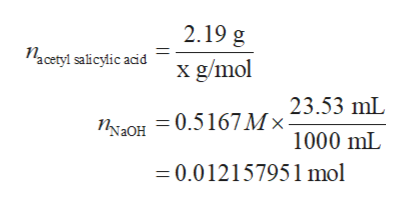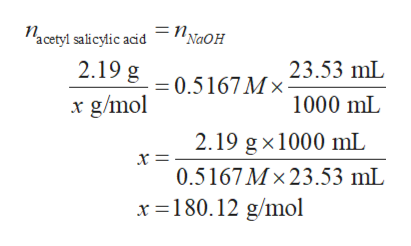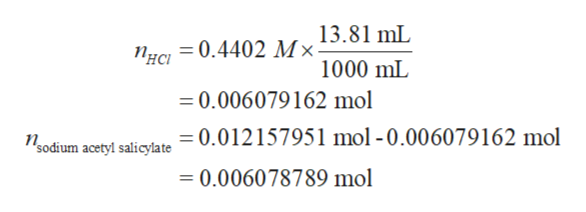# The active ingredient in aspirin is acetylsalicylic acid. A 2.19 g sample of acetylsalicylic acid required 23.53 mL of 0.5167 M NaOH for a complete reaction. The addition of 13.81 mL of 0.4402 M HCl to the flask containing the aspirin and the sodium hydroxide produced a mixture with pH = 3.48. Find the molar mass (in g/mol) of acetylsalicylic acid and its Ka value. Acetylsalicylic acid is a monoprotic acid.

Question
61 views
The active ingredient in aspirin is acetylsalicylic acid. A 2.19 g sample of acetylsalicylic acid required 23.53 mL of 0.5167 M NaOH for a complete reaction. The addition of 13.81 mL of 0.4402 M HCl to the flask containing the aspirin and the sodium hydroxide produced a mixture with pH = 3.48. Find the molar mass (in g/mol) of acetylsalicylic acid and its Ka value. Acetylsalicylic acid is a monoprotic acid.

check_circle

Step 1

The number of moles of acetylic salicylic acid and sodium hydroxide reacted are equal because acetylsalicylic acid is monoprotic and complete neutralization reaction takes place. Let the molar mass of acetylsalicylic acid is x g/mol. The number of moles of acetylic salicylic acid and sodium hydroxide is calculated using the equation below.help_outlineImage Transcriptionclose2.19 g Nacetyi salicylic acid x g/mol 23.53 mL = 0.5167 M ×- NN2OH 1000 mL = 0.012157951 mol fullscreen
Step 2

The molar mass of acetylsalicylic acid is calculated by equating the moles of acetylic salicylic acid and sodium hydroxide:help_outlineImage Transcriptionclose=N NaOH Nacetyl salicylic acid 2.19 g 23.53 mL = 0.5167 M × x g/mol 1000 mL 2.19 g x1000 mL 0.5167 M × 23.53 mL x =180.12 g/mol fullscreen
Step 3

The number of moles of acetylsalicylate formed is 0.012 mole. When hydrochloric acid will be added to formed sodium acetylsalicylate it will convert bac...help_outlineImage Transcriptionclose13.81 mL Мx 1000 mL Пнс — 0.4402 = 0.006079162 mol = 0.012157951 mol - 0.006079162 mol Проdium acetyl salicylate = 0.006078789 mol fullscreen

### Want to see the full answer?

See Solution

#### Want to see this answer and more?

Solutions are written by subject experts who are available 24/7. Questions are typically answered within 1 hour.*

See Solution
*Response times may vary by subject and question.
Tagged in

### Chemistry